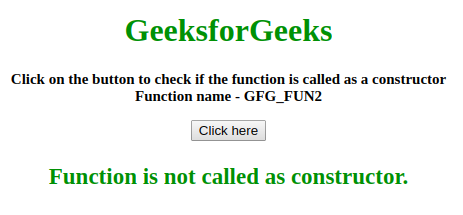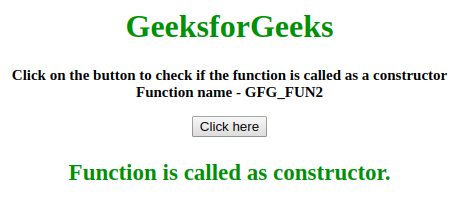# How to detect if a function is called as constructor?

The problem is to identify where the function call is a constructor call or not.

Approach 1:

• Use the instanceof property.
• If the current instance is the instance of function then this is a constructor call.
• Else, this is a general function call.

Example: This example implements the above approach.

 ` ` `<``html``> ` ` `  `<``head``> ` `    ``<``title``> ` `        ``How to detect if a function is called ` `        ``as constructor in JavaScript ? ` `    `` ` ` ` ` `  `<``body` `style``=``"text-align:center;"``> ` `     `  `    ``<``h1` `style``=``"color:green;"``>  ` `        ``GeeksForGeeks  ` `    `` ` `     `  `    ``<``p` `id``=``"GFG_UP"` `style``= ` `        ``"font-size: 15px; font-weight: bold;"``> ` `    `` ` `     `  `    ``<``button` `onclick``=``"gfg_Run()"``> ` `        ``Click here ` `    `` ` `     `  `    ``<``p` `id``=``"GFG_DOWN"` `style``= ` `        ``"font-size: 23px; font-weight: bold; color: green; "``> ` `    `` ` `     `  `    ``<``script``> ` `        ``var el_up = document.getElementById("GFG_UP"); ` `        ``var el_down = document.getElementById("GFG_DOWN"); ` `         `  `        ``el_up.innerHTML = "Click on the button to check if the " ` `                    ``+ "function is called as a constructor<``br``>" ` `                    ``+ "Function name - GFG_FUN2"; ` ` `  `        ``function GFG_FUN2(val) { ` `            ``var temp = false; ` `             `  `            ``if (this instanceof GFG_FUN2 &&  ` `                    ``!this.__previouslyConstructedByVal) { ` `                ``temp = true; ` `                ``this.__previouslyConstructedByVal = true; ` `            ``} ` `             `  `            ``return temp; ` `        ``} ` ` `  `        ``function gfg_Run() { ` `             `  `            ``// Function call  ` `            ``var temp = GFG_FUN2(); ` `             `  `            ``if (temp == true) { ` `                ``el_down.innerHTML =  ` `                        ``"Function is called as constructor."; ` `            ``}  ` `            ``else { ` `                ``el_down.innerHTML =  ` `                        ``"Function is not called as constructor."; ` `            ``} ` `        ``} ` `    `` ` ` ` ` `  ` `

Output:

• Before clicking on the button:• After clicking on the button:Approach 2:

• Use the .constructor property.
• If this.constructor is equal to the function name then this is a constructor call.
• Else, this is a general function call.

Example: This example illustrate the approach discussed above.

 ` ` `<``html``> ` ` `  `<``head``> ` `    ``<``title``> ` `        ``Detect function is called as constructor ` `    `` ` ` ` ` `  `<``body` `style``=``"text-align:center;"` `id``=``"body"``> ` `    ``<``h1` `style``=``"color:green;"``>   ` `            ``GeeksforGeeks   ` `        `` ` `    ``<``p` `id``=``"GFG_UP"` `style``=``"font-size: 15px; font-weight: bold;"``> ` `    `` ` `    ``<``button` `onclick``=``"gfg_Run()"``> ` `        ``Click here ` `    `` ` `    ``<``p` `id``=``"GFG_DOWN"`  `       ``style``=``"font-size: 23px; font-weight: bold; color: green; "``> ` `    `` ` `    ``<``script``> ` `        ``var el_up = document.getElementById("GFG_UP"); ` `        ``var el_down = document.getElementById("GFG_DOWN"); ` `        ``el_up.innerHTML = "Click on the button to check if the " + ` `                          ``"function is called as a constructor<``br``>" +  ` `                          ``"Function name - GFG_FUN2"; ` ` `  `        ``function GFG_FUN2(val) { ` `            ``var temp = false; ` `            ``if (this.constructor == GFG_FUN2) { ` `                ``temp = true; ` `            ``} ` `            ``if (temp == true) { ` `                ``el_down.innerHTML = "Function is called as constructor."; ` `            ``} else { ` `                ``el_down.innerHTML = "Function is not called as constructor."; ` `            ``} ` `        ``} ` ` `  `        ``function gfg_Run() { ` `            ``new GFG_FUN2(); //Call to the function. ` `        ``} ` `    `` ` ` ` ` `  ` `

Output:

• Before clicking on the button:• After clicking on the button:My Personal Notes arrow_drop_upCheck out this Author's contributed articles.

If you like GeeksforGeeks and would like to contribute, you can also write an article using contribute.geeksforgeeks.org or mail your article to contribute@geeksforgeeks.org. See your article appearing on the GeeksforGeeks main page and help other Geeks.

Please Improve this article if you find anything incorrect by clicking on the "Improve Article" button below.